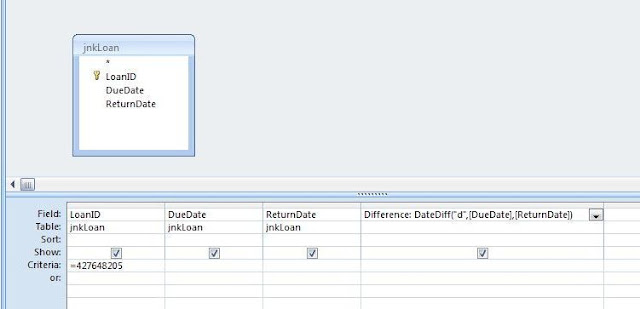## Friday, 12 August 2011

### Calculating Date Difference: Using the DateDiff Function

Suppose you want to calculate the difference between two dates.  An example of this might be a Library Management System: somebody returns an overdue library book, and receives a fine based on the number of days it happens to be late.  Our two dates in this instance would be the DueDate and the actual DateReturned.  To calculate the difference between these two dates we may use the DateDiff Function.

We could use the DateDiff Function as a Calculated Control (just put an = sign in front of it and enter the function as the textbox's CONTROL SOURCE), or within a VBA Code Module.  In this example, however, we shall use it within an Access Query.

Let's take a look at how the DateDiff Function is constructed:

`DateDiff("interval", FirstDate, SecondDate)`

As you can see, the function passes three parameters: these are "Interval", FirstDate, and SecondDate.  The interval parameter allows us to specify whether we want the function to return the difference in, for example, days, weeks, or years.  In our case we are interested in how many days a book is overdue, so we enter "d" as a string value. (Had we wanted the interval in weeks, we would have entered the parameter as "ww"; or years as "yyyy".  There are also a number of other options available such as quarter: "q"; hours: "h"; and minutes: "n").  The FirstDate and SecondDate parameter's refer to the two dates between which the difference is to be calculated.  In our case, these dates are contained in the DueDate and ReturnDate fields of a table called jnkLoan. As such, we would construct the DateDiff Function as follows:

`DateDiff("d", DueDate, ReturnDate)`
```
```
Incidentally if the book was returned early, ie the DueDate is later than the ReturnDate, the function would return the Date Difference as a negative value.  As such, the order in which the two date parameters are entered will make a difference to the return value of the function. It is the same principle as the subtraction of a smaller number from a larger number, and vice versa. With the DateDiff Function, the value of the first date is "subtracted" from that of the second, to return the date difference which we defined in the interval parameter.

So lets take a look at how we may use this function in the context of an Access Query:Figure 1: The DateDiff Function is used in the last column on the right.

For the sake of simplicity I have used a table with just three fields - LoanID, DueDate, and ReturnDate.  However, as you can see from the screen shot above, our query has four columns.  The first three columns contain the fields of our jnkLoan table, but the last column on the right is not bound to any field; it is actually a calculation based on the DateDiff Function that we constructed above. To use the function within this query we have just had to choose an alias for the column - ie a name we make up to refer to the column.  In this example, we have used an alias called Difference.  A colon is then used to separate the alias from our DateDiff Function.

Another thing worth mentioning is how our DateDiff Function refers to the DueDate and ReturnDate fields of the jnkLoan table.  As such our Query bases the DateDiff calculation on the date values contained in the relevant record (ie that which matches the criteria set in the LoanID column) of this table.  Here is the result:Figure 2: Results of the Query using the DateDiff Function. The result of the DateDiff calculation is displayed in the last column on the right.
Our query criteria selected a record containing the LoanID 427648205.  The DueDate for this record was the 08/08/2011 and the ReturnDate is 12/08/2011.  Our DateDiff Function, displayed in the last column on the right, has calculated the book is being returned four days overdue.

1.How do I use the DateDiff in an access form?

2.nevermind...got it

3.Hey! You were born a Teacher! Have been looking for this information but it hasn't been clearly explained. I would like to befriend you because i will learn alot from you.

1.Hi Yamiko

What was it you didn't understand in relation to this post?